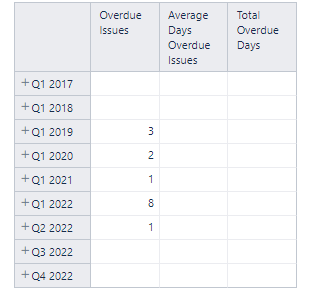# Average Time of Overdue Issues

We are currently using this formula to get the Overdue issues based on the due date:
NonZero(count(
Filter(Descendants([Issue].CurrentMember,[Issue].[Issue]),
[Measures].[Issues with due date]>0 and
isempty([Measures].[Issues not resolved]) and
DateCompare([Measures].[Issue due date],Now())<0
)
))

Now we want to get the average time/days the issues are overdue based on the formula above from their due date up to the present. Can you please help us with the formula?

Hi @jeno,

I can’t test this on my side since your formula uses custom measures, but here is what I think would work for you.

First, create a Total Overdue Days measure like this:

``````CASE WHEN
[Measures].[Overdue Issue (your calculated measure here)] > 0
THEN
-- total time from issue due date till now
DateDiffDays(
[Issue].CurrentHierarchyMember.get('Due date'),
[Issue].CurrentHierarchyMember.Get('Now()')
)
``````

Then, you could create your average

``````CASE WHEN [Measures].[Overdue Issue (your calculated measure here)] > 0 THEN
[Measures].[Total Overdue Days] / [Measures].[Overdue Issue (your calculated measure here)]
END
``````

Let me know how this works for you!
Marilou

Hi Marilou,

I created the formula based on yours, however, I could not get the data out of it. See results below.Hi @jeno

You were on the right track with the initial formula: iterating through issues is the way to go to get the average (find the overdue time for each issue and calculate the average).
For that, instead of Count(), you would use Avg(). For the average calculation, you need a set of issues and a numerical expression:

1. the set would be the same as in your Count() calculation (with a few slight changes),
2. the numerical expression would be the DateDiffDays() calculation between the due date and today, shared by @Marilou

So, the final calculation could be the following:

``````Avg(
Filter(
Descendants([Issue].CurrentMember,[Issue].[Issue]),
Isempty([Measures].[Issue resolution date]) and
DateCompare([Measures].[Issue due date],Now())<0 and
([Measures].[Issues with due date],
[Time].CurrentHierarchy.DefaultMember)>0),
DateDiffDays(
[Issue].CurrentHierarchyMember.get('Due date'),
Now()
)
)

``````

Remember to set decimal formatting!

Best,
Ilze / support@eazybi.com

1 Like